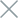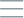• Home
• Tags
• Series
•# Random Search Algorithm in PHP: Explained with Example

The Random Search Algorithm is a significant approach in PHP programming, used to explore a search space by randomly selecting solutions and evaluating them. The goal of this algorithm is to search for potential solutions within the search space.

## How the Random Search Algorithm Works

The Random Search Algorithm starts by randomly selecting a set of solutions from the search space. It then evaluates the quality of the solutions using an evaluation function. The algorithm may repeat this process multiple times to search for potentially better solutions.

• Wide Exploration Space: This algorithm has the capability to explore a wide range of the search space by evaluating various solutions.
• Easy to Implement: The Random Search Algorithm is generally easy to implement and doesn't require extensive expertise.

• Lack of Global Optimization Guarantee: This algorithm may not find the globally optimal solution and tends to focus on solutions that are closer to the initial position.
• Time-Consuming: The Random Search Algorithm can be time-consuming as it needs to evaluate multiple solutions.

## Example and Explanation

Consider an example of searching for prime numbers within a specific range using the Random Search Algorithm in PHP.

``````function randomSearch(\$min, \$max, \$numTrials) {
for (\$i = 0; \$i < \$numTrials; \$i++) {
\$randomNumber = rand(\$min, \$max);
if (isPrime(\$randomNumber)) {
return \$randomNumber;
}
}
return "No prime found in the given range.";
}

function isPrime(\$num) {
if (\$num <= 1) {
return false;
}
for (\$i = 2; \$i <= sqrt(\$num); \$i++) {
if (\$num % \$i === 0) {
return false;
}
}
return true;
}

\$min = 100;
\$max = 1000;
\$numTrials = 50;

In this example, we use the Random Search Algorithm to find a prime number within the range from 100 to 1000. The algorithm randomly selects numbers from this range and checks if they are prime using the `isPrime` function. The result is a randomly found prime number within the specified range.